In the boiling water reactors (BWRs), reactor cooling water is maintained in high purity condition by controlling of a deionizing and deaerating apparatus, however H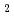Ocontains by water radiolysis. In order to determine the corrosive condition in high-temperature pure water containing HO, the electrochemical impedance spectroscopy was performed in this study. To simulate BWR condition precisely, the measurements were performed without any electrolyte. The obtained impedance responses were changed with the HOconcentration. The solution resistance and polarization resistance were determined by the equivalent circuit analyses. The conductivity was determined by the obtained solution resistance and the calculation of the current flow between the working electrode and the counter electrode by the 3-demensional finite element method. The value, 4.4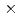10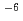S/cm, was obtained as the conductivity of the pure water at 288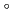C. The reciprocal of the obtained polarization resistance increased in proportion with HOconcentration. This indicates that the corrosion current density was determined by the diffusion limiting current density of HO. The diffusion coefficient of HOat 288C was determined using the relationship between the reciprocal of the polarization resistance and HOconcentration. The obtained diffusion coefficient was 1.510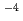cm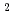/s. This is about twice larger than that of the reported value of O.